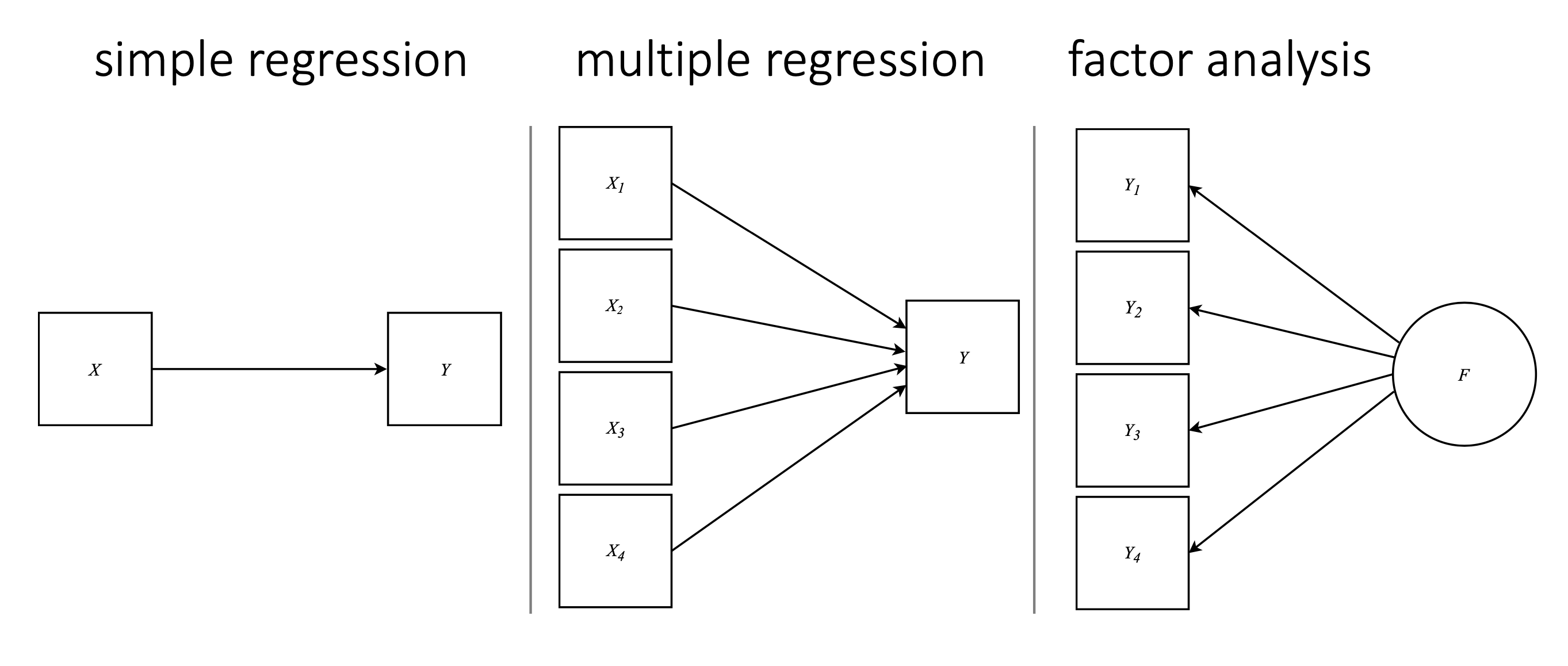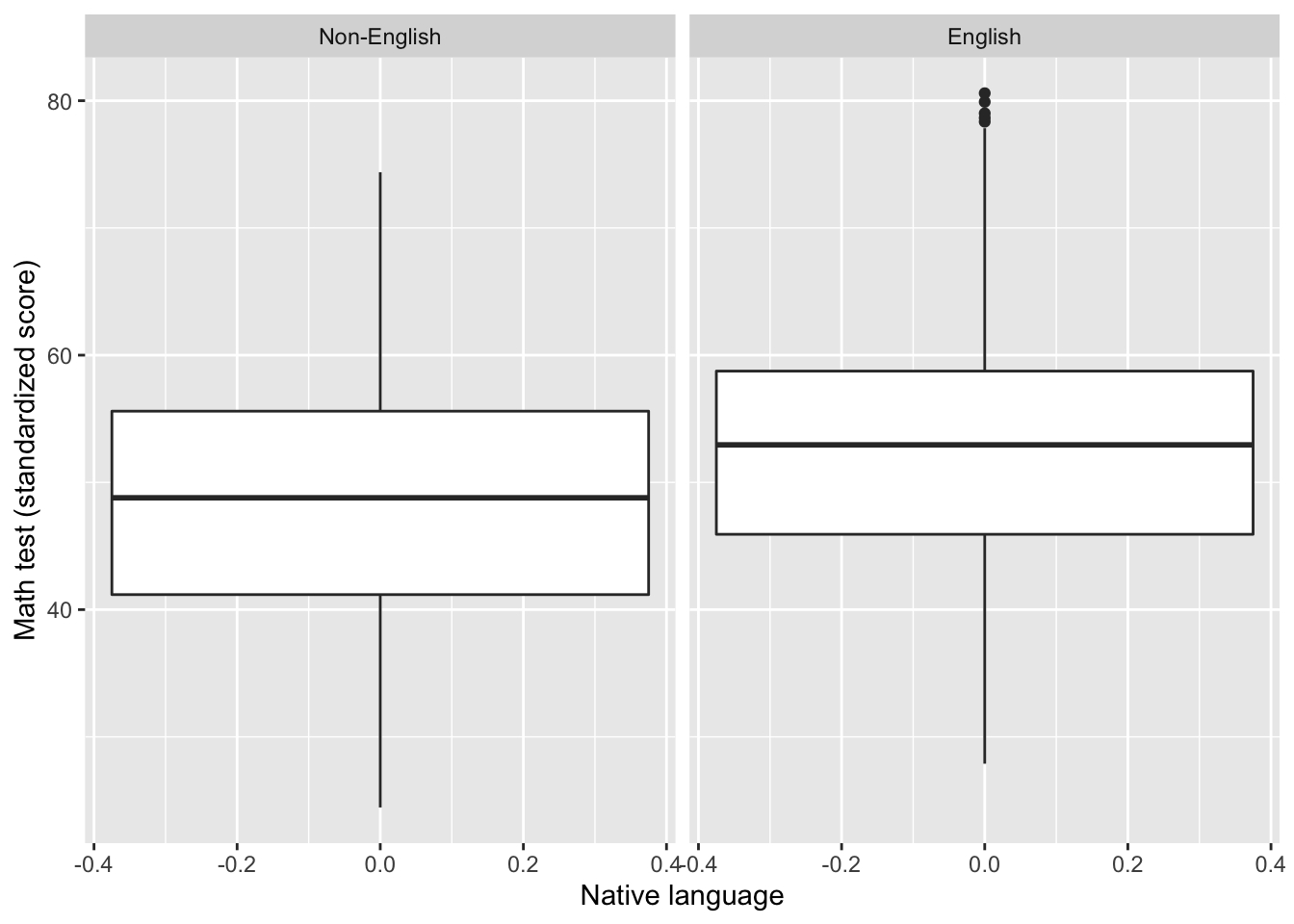#### $$\color{purple}{\text{Tools for reproducibility:}}$$

{MplusAutomation} package Current capabilities supporting full SEM modeling High flexibility
R Project Unbreakable file paths & neatness Reproducibility (kindness to your future self)
{tidyverse} package Intuitive/descriptive function names Accessibility to new users
{here} package Unbreakable/consistent file paths across OS Reproducibility (for Science’s sake!)
{haven} package View-able metadata in R from SPSS data-files Getting to know your measures
{ggplot2} package Clear, customizable, reproducible figures Publication quality data visualizations
pipe operator (%>%) notation Ease of reading/writing scripts e.g., first() %>% and_then() %>% and_finally()

### $$\color{purple}{\text{Creating a version-controlled R-Project by downloading repository from Github}}$$

Download ropository here: $$\color{blue}{\text{https://github.com/garberadamc/SEM-Lab1}}$$

#### Create a class folder (to save labs and assignments)

1. click “NEW PROJECT” (upper right corner of window)
2. choose option Version Control
3. choose option Git
4. paste the repository web URL path coppied from the clone or download button on the repo page
5. choose location of the R-Project ($$\color{red}{\text{too many nested folders will result in filepath error}}$$)

#### Create sub-folders within the project folder. In R-studio under the files pane …

1. click “New Folder” and name folder “data”
2. click “New Folder” and name folder “mplus_files”
3. click “New Folder” and name folder “figures”

Install the “rhdf5” package to read gh5 files

if (!requireNamespace("BiocManager", quietly = TRUE))
install.packages("BiocManager")
BiocManager::install("rhdf5")

### $$\color{purple}{\text{Load packages}}$$

library(MplusAutomation)
library(haven)
library(rhdf5)
library(tidyverse)
library(here)
library(corrplot)
library(kableExtra)
library(reshape2)
library(janitor)
library(ggridges)
library(DiagrammeR)
library(semPlot)
library(sjPlot)

Keyboard shortcuts

• ALT + DASH(-) = <-
• SHIFT + CONTROL = %>%

### $$\color{purple}{\text{Read in SPSS data}}$$

spss_data <- read_spss(here("data", "els_sub1_spss.sav")) %>%
janitor::clean_names() # makes all variable names lowercase

### $$\color{purple}{\text{Preparations: subset, rename, and reorder columns}}$$

1. subset: select columns in 3 ways, remove columns with (-), select by index number, and select by column name
2. rename: change variable names to be descriptive and within the Mplus 8 character limit
3. reorder: this makes it easy to choose sequential variables for {MplusAutomation}
spss_sub0 <- spss_data %>%
select(-stu_id,   -sch_id,   -byrace,
-byparace, -byparlng, -byfcomp,
-bypared,  -bymothed, -byfathed,
-bysctrl,  -byurban,  -byregion)

### $$\color{purple}{\text{Select the first 9 columns (by index) and select the next 17 columns (by name)}}$$

spss_sub1 <- spss_sub0 %>%
select(1:9,
bys20a, bys20h, bys20j, bys20k, bys20m, bys20n,
bys21b, bys21d, bys22a, bys22b, bys22c, bys22d,
bys22e, bys22g, bys22h, bys24a, bys24b) %>%
rename("stu_exp" = "bystexp",   # "NEW_NAME" = "OLD_NAME"
"par_asp" = "byparasp",
"mth_test" = "bytxmstd",
"rd_test" = "bytxrstd",
"freelnch" = "by10flp",
"stu_tch" = "bys20a",
"putdownt" = "bys20h",
"unsafe" = "bys20j",
"disrupt" = "bys20k",
"gangs" = "bys20m",
"rac_fght" = "bys20n",
"fair" = "bys21b",
"strict" = "bys21d",
"stolen" = "bys22a",
"drugs" = "bys22b",
"t_hurt" = "bys22c",
"p_fight" = "bys22d",
"hit" = "bys22e",
"damaged" = "bys22g",
"bullied" = "bys22h",
"late" = "bys24a",
"skipped" = "bys24b")

### $$\color{purple}{\text{More housekeeping: reorder columns }}$$

spss_sub2 <-  spss_sub1 %>%
select(
bystlang,                                        # dichotomous (yes,no)
freelnch, byincome,                              # ordinal (binned, continuous scale)
stolen, t_hurt, p_fight, hit, damaged, bullied,  # ordinal frequency (3-point)
unsafe, disrupt, gangs, rac_fght,                # ordinal Likert (4-point scale)
late, skipped,                                   # ordinal frequency (4-point scale)
mth_test, rd_test)                               # continuous (standardized test scores)

### $$\color{purple}{\text{Make a codebook including metadata using {sjPlot}}}$$

sjPlot::view_df(spss_sub2)

### $$\color{purple}{\text{Types of data for different tasks}}$$

• SAV (e.g., spss_data.sav): this data format is for SPSS files & contains variable labels (meta-data)
• CSV (e.g., r_ready_data.csv): this is the preferable data format for reading into R (no labels)
• DAT (e.g., mplus_data.dat): this is the data format used to read into Mplus (no column names or strings)

NOTE: Mplus also accepts TXT formatted data (e.g., mplus_data.txt)

### $$\color{purple}{\text{Converting data between 3 formats: writing and reading data}}$$

Write a CSV datafile (preferable format for reading into R, with SPSS labels removed)

write_csv(spss_sub2, here("data", "els_sub6_data.csv"))

Write a SPSS datafile (preferable format for reading into SPSS, labels are preserved)

write_sav(spss_sub2, here("data", "els_sub6_data.sav"))

Read the unlabeled data back into R

tidy_data <- read_csv(here("data", "els_sub6_data.csv"))

Write a DAT datafile for Mplus (this function removes header row & converts missing values to non-string)

prepareMplusData(tidy_data, here("data", "els_sub6_data.dat"))

### $$\color{purple}{\text{Make a tribble table}}$$

var_table <- tribble(
~"Name",    ~"Labels",                                      ~"Value Labels (limit)",
#-----------|-----------------------------------------------|--------------------------------------|,
"bystlang" , "Whether English is students native language" ,"0=No, 1=Yes",
"freelnch" , "Grade 10 percent free lunch-categorical"     ,"0=0-5%, 7=76-100%",
"byincome" , "Total family income from all sources 2001"   ,"1=None, 13=\$200,001 or more",
"stolen"   , "Had something stolen at school"              ,"1=Never, 3=More than twice",
"t_hurt"   , "Someone threatened to hurt 10th grader at school","1=Never, 3=More than twice",
"p_fight"  , "Got into a physical fight at school"         ,"1=Never, 3=More than twice"  ,
"hit"      , "Someone hit 10th grader"                     ,"1=Never, 3=More than twice"  ,
"damaged"  , "Someone damaged belongings"                  ,"1=Never, 3=More than twice"  ,
"bullied"  , "Someone bullied or picked on 10th grader"    ,"1=Never, 3=More than twice"  ,
"unsafe"   , "Does not feel safe at this school"           ,"1=Strongly agree, 4=Strongly disagree"  ,
"disrupt"  , "Disruptions get in way of learning"          ,"1=Strongly agree, 4=Strongly disagree"  ,
"gangs"    , "There are gangs in school"                   ,"1=Strongly agree, 4=Strongly disagree"  ,
"rac_fght" , "Racial-ethnic groups often fight"            ,"1=Strongly agree, 4=Strongly disagree"  ,
"late"     , "How many times late for school"              ,"1=Never, 4=10 or more times"  ,
"skipped"  , "How many times cut-skip classes"             ,"1=Never, 4=10 or more times"  ,
"mth_test" , "Math test standardized score"                ,"0-100"  ,
"rd_test"  , "Reading test standardized score"             ,"0-100"  ,
)

var_table %>%
kable("latex", booktabs = T, linesep = "") %>%
kable_styling(latex_options = c("striped"),
full_width = F,
position = "left")

### $$\color{purple}{\text{Take a look at the data - some practice with {ggplot2}}}$$

Make a facetted box plot

# some formatting, add labels to bystlang for plot
tidy_data <- tidy_data %>%
mutate(
bystlang = factor(bystlang,
labels = c(0 = "Non-English", 1 = "English")))

ggplot(data=drop_na(tidy_data), aes(y=mth_test)) +
geom_boxplot() +
facet_wrap(~bystlang) +
labs(x = "Native language",
y = "Math test (standardized score)")Make a density plot

  ggplot(data=drop_na(tidy_data), aes(x=mth_test)) +
geom_density(aes(fill = bystlang),
color = NA,
show.legend = FALSE) +
facet_wrap(~bystlang) +
theme_light()